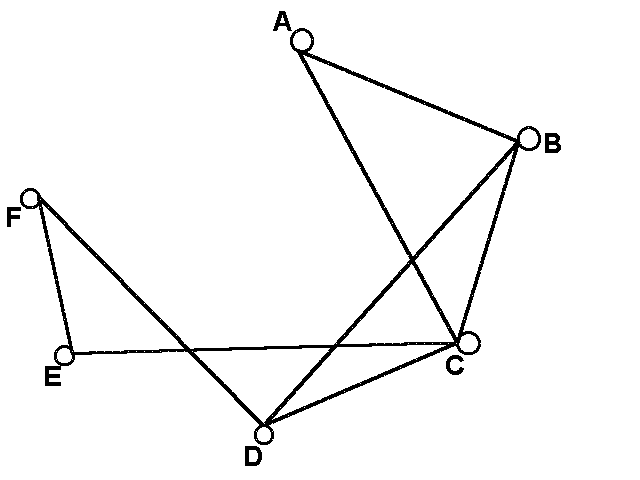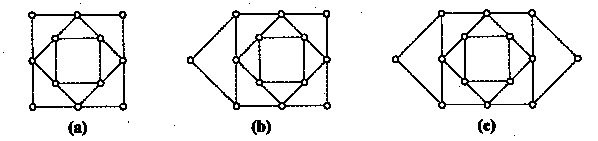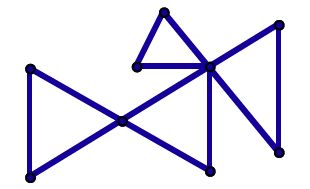# 6.5: Exercises

•• Maxie Inigo, Jennifer Jameson, Kathryn Kozak, Maya Lanzetta, & Kim Sonier
• Coconino Community College
$$\newcommand{\vecs}{\overset { \rightharpoonup} {\mathbf{#1}} }$$ $$\newcommand{\vecd}{\overset{-\!-\!\rightharpoonup}{\vphantom{a}\smash {#1}}}$$$$\newcommand{\id}{\mathrm{id}}$$ $$\newcommand{\Span}{\mathrm{span}}$$ $$\newcommand{\kernel}{\mathrm{null}\,}$$ $$\newcommand{\range}{\mathrm{range}\,}$$ $$\newcommand{\RealPart}{\mathrm{Re}}$$ $$\newcommand{\ImaginaryPart}{\mathrm{Im}}$$ $$\newcommand{\Argument}{\mathrm{Arg}}$$ $$\newcommand{\norm}{\| #1 \|}$$ $$\newcommand{\inner}{\langle #1, #2 \rangle}$$ $$\newcommand{\Span}{\mathrm{span}}$$ $$\newcommand{\id}{\mathrm{id}}$$ $$\newcommand{\Span}{\mathrm{span}}$$ $$\newcommand{\kernel}{\mathrm{null}\,}$$ $$\newcommand{\range}{\mathrm{range}\,}$$ $$\newcommand{\RealPart}{\mathrm{Re}}$$ $$\newcommand{\ImaginaryPart}{\mathrm{Im}}$$ $$\newcommand{\Argument}{\mathrm{Arg}}$$ $$\newcommand{\norm}{\| #1 \|}$$ $$\newcommand{\inner}{\langle #1, #2 \rangle}$$ $$\newcommand{\Span}{\mathrm{span}}$$$$\newcommand{\AA}{\unicode[.8,0]{x212B}}$$

1. Answer the following questions based on the graph below.1. What are the vertices?
2. Is this graph connected?
3. What is the degree of vertex C?
4. Edge FE is adjacent to which edges?
5. Does this graph have any bridges?
1. Answer the following questions based on the graph below.1. What are the vertices?
2. What is the degree of vertex u?
3. What is the degree of vertex s?
4. What is one circuit in the graph?
1. Draw a spanning subgraph in the graph below.1. Find the minimum spanning tree in the graph below using Kruskal’s Algorithm.1. Find the minimum spanning tree in the graph below using Kruskal’s Algorithm.1. Find an Euler Path in the graph below.1. Find an Euler circuit in the graph below.1. Which graphs below have Euler Paths? Which graphs have Euler circuits?1. Highlight an Euler circuit in the graph below.1. For each of the graphs below, write the degree of each vertex next to each vertex.1. Circle whether each of the graphs below has an Euler circuit, an Euler path, or neither.Euler circuit Euler path Neither Euler circuit Euler path Neither Euler circuit Euler path Neither
1. How many Hamilton circuits does a complete graph with 6 vertices have?
1. Suppose you need to start at A, visit all three vertices, and return to the starting point A. Using the Brute Force Algorithm, find the shortest route if the weights represent distances in miles.1. If a graph is connected and __________________, the graph will have an Euler circuit.
1. the graph has an even number of vertices
2. the graph has an even number of edges
3. the graph has all vertices of even degree
4. the graph has only two odd vertices
1. Starting at vertex A, use the Nearest-Neighbor Algorithm to find the shortest route if the weights represent distances in miles.1. Find a Hamilton circuit using the Repetitive Nearest-Neighbor Algorithm.1. Find a Hamilton circuit using the Cheapest-Link Algorithm.1. Which is a circuit that traverses each edge of the graph exactly once?

A. Euler circuit b. Hamilton circuit c. Minimum Spanning Tree

1. Which is a circuit that traverses each vertex of the graph exactly once?

A. Euler circuit b. Hamilton circuit c. Minimum Spanning Tree

1. For each situation, would you find an Euler circuit or a Hamilton Circuit?
1. The department of Public Works must inspect all streets in the city to remove dangerous debris.
2. Relief food supplies must be delivered to eight emergency shelters located at different sites in a large city.
3. The Department of Public Works must inspect traffic lights at intersections in the city to determine which are still working.
4. An insurance claims adjuster must visit 11 homes in various neighborhoods to write reports.

This page titled 6.5: Exercises is shared under a CC BY-SA 4.0 license and was authored, remixed, and/or curated by Maxie Inigo, Jennifer Jameson, Kathryn Kozak, Maya Lanzetta, & Kim Sonier via source content that was edited to the style and standards of the LibreTexts platform; a detailed edit history is available upon request.